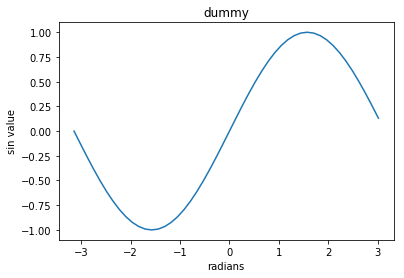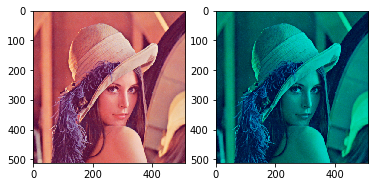# Python

Python

We will use the Python programming language, with a few popular libraries (numpy, matplotlib). If you have previous knowledge in Matlab, check out the numpy for Matlab users page. The section below will serve as a quick introduction on Numpy and some other libraries.

Setup Python environment

We can install Anaconda from the links given below. You can setup your environment using Anaconda for Python 2.7 or 3.6.

Please use nbconvert tool. This can be installed from instructions given on:
nbconvert: "conda install nbconvert" (or http://nbconvert.readthedocs.io/en/latest/install.html)
The above link also gives instructions for installing Pandoc and Latex for different OS. Please follow those instructions as installing these might be required for nbconvert.

# Get started with Numpy

Numpy is the fundamental package for scientific computing with Python. It provides a powerful N-dimensional array object and functions for working with these arrays.

## Arrays

import numpy as np

v = np.array([1, 0, 0])        # a 1d array
print("1d array")
print(v)
print(v.shape)                 # print the size of v
v = np.array([, , ])  # a 2d array
print("\n2d array")
print(v)
print(v.shape)                 # print the size of v, notice the difference
v = v.T                        # transpose of a 2d array

m = np.zeros([2, 3])           # a 2x3 array of zeros
v = np.ones([1, 3])            # a 1x3 array of ones
m = np.eye(3)                  # identity matrix
v = np.random.rand(3, 1)       # random matrix with values in [0, 1]
m = np.ones(v.shape) * 3       # create a matrix from shape

1d array
[1 0 0]
(3,)

2d array
[

]
(3, 1)


### Array indexing

import numpy as np

m = np.array([[1, 2, 3], [4, 5, 6]])  # create a 2d array with shape (2, 3)
print("Access a single element")
print(m)
print(m[:2,1:3])

print(m[0, 2])                        # access an element
m[0, 2] = 252                         # a slice of an array is a view into the same data;
print("\nModified a single element")
print(m)                              # this will modify the original array

print("\nAccess a subarray")
print(m[1, :])                        # access a row (to 1d array)
print(m[1:, :])                       # access a row (to 2d array)
print("\nTranspose a subarray")
print(m[1, :].T)                      # notice the difference of the dimension of resulting array
print(m[1:, :].T)                     # this will be helpful if you want to transpose it later

# Boolean array indexing
# Given a array m, create a new array with values equal to m
# if they are greater than 0, and equal to 0 if they less than or equal 0

m = np.array([[3, 5, -2], [5, -1, 0]])
n = np.zeros(m.shape)
n[m > 0] = m[m > 0]
print("\nBoolean array indexing")
print(n)

Access a single element
[[1 2 3]
[4 5 6]]
[[2 3]
[5 6]]
3

Modified a single element
[[  1   2 252]
[  4   5   6]]

Access a subarray
[4 5 6]
[[4 5 6]]

Transpose a subarray
[4 5 6]
[

]

Boolean array indexing
[[ 3.  5.  0.]
[ 5.  0.  0.]]


### Operations on array

Elementwise Operations

import numpy as np

a = np.array([[1, 2, 3], [2, 3, 4]], dtype=np.float64)
print(a * 2)                                            # scalar multiplication
print(a / 4)                                            # scalar division
print(np.round(a / 4))
print(np.power(a, 2))
print(np.log(a))

b = np.array([[5, 6, 7], [5, 7, 8]], dtype=np.float64)
print(a + b)                                            # elementwise sum
print(a - b)                                            # elementwise difference
print(a * b)                                            # elementwise product
print(a / b)                                            # elementwise division

[[ 2.  4.  6.]
[ 4.  6.  8.]]
[[ 0.25  0.5   0.75]
[ 0.5   0.75  1.  ]]
[[ 0.  0.  1.]
[ 0.  1.  1.]]
[[  1.   4.   9.]
[  4.   9.  16.]]
[[ 0.          0.69314718  1.09861229]
[ 0.69314718  1.09861229  1.38629436]]
[[  6.   8.  10.]
[  7.  10.  12.]]
[[-4. -4. -4.]
[-3. -4. -4.]]
[[  5.  12.  21.]
[ 10.  21.  32.]]
[[ 0.2         0.33333333  0.42857143]
[ 0.4         0.42857143  0.5       ]]


Vector Operations

import numpy as np

a = np.array([[1, 2], [3, 4]])
print("sum of array")
print(np.sum(a))                # sum of all array elements
print(np.sum(a, axis=0))        # sum of each column
print(np.sum(a, axis=1))        # sum of each row
print("\nmean of array")
print(np.mean(a))               # mean of all array elements
print(np.mean(a, axis=0))       # mean of each column
print(np.mean(a, axis=1))       # mean of each row

sum of array
10
[4 6]
[3 7]

mean of array
2.5
[ 2.  3.]
[ 1.5  3.5]


Matrix Operations

import numpy as np

a = np.array([[1, 2], [3, 4]])
b = np.array([[5, 6], [7, 8]])
print("matrix-matrix product")
print(a.dot(b))                 # matrix product
print(a.T.dot(b.T))

x = np.array([1, 2])
print("\nmatrix-vector product")
print(a.dot(x))                 # matrix / vector product

matrix-matrix product
[[19 22]
[43 50]]
[[23 31]
[34 46]]

matrix-vector product
[ 5 11]


### SciPy image operations

SciPy builds on the Numpy array object and provides a large number of functions useful for scientific and engineering applications. We will show some examples of image operation below which are useful for this class.

from imageio import imread, imsave
import numpy as np

img = imread('Lenna.png')  # read an JPEG image into a numpy array
print(img.shape)                          # print image size and color depth

img_gb = img * np.array([0., 1., 1.])     # leave out the red channel
imsave('Lenna_gb.png', img_gb.astype(np.uint8))

(512, 512, 3)


### Matplotlib

Matplotlib is a plotting library. We will use it to show result in this assignment.

# this line prepares IPython for working with matplotlib
%matplotlib inline

import numpy as np
import matplotlib.pyplot as plt
import math

x = np.arange(-24, 24) / 24. * math.pi
plt.plot(x, np.sin(x))
plt.ylabel('sin value')
plt.title('dummy')

plt.show()# images and subplot
import numpy as np
import matplotlib.pyplot as plt

plt.subplot(1, 2, 1)  # first plot
plt.imshow(img1)

print(img1.shape)

plt.subplot(1, 2, 2) # second plot
plt.imshow(img2, cmap = 'gray')
plt.show()

(512, 512, 3)This breif overview introduces many basic functions from a few popular libraries, but is far from complete. Check out the documentations for Numpy, Scipy and Matplotlib to find out more.

Q.E.D.

To baldly -_- go no man has gone before